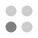• 14,522 Trophies Earned
• 709 Players Tracked
• 21 Total Trophies
• 210 Obtainable EXP
• 1,170 Points
• 679 Platinum Club
• 679 100% Club

### Energy Cycle Edge Trophies

•96.19%
•Solved first puzzle100.00%
•Puzzles 2, 3 and 4 solved99.72%
•Puzzles 5, 6 and 7 solved99.15%
•Puzzles 8, 9 and 10 solved99.01%
•Puzzles 11, 12 and 13 solved98.45%
•Puzzles 14, 15 and 16 solved97.46%
•Puzzles 17, 18 and 19 solved97.32%
•Puzzles 20, 21 and 22 solved97.18%
•Puzzles 23, 24 and 25 solved96.90%
•Puzzles 26, 27 and 28 solved96.90%
•Puzzles 29, 30 and 31 solved96.76%
•Puzzles 32, 33 and 34 solved96.33%
•Puzzles 35 and 36 solved96.33%
•Puzzles 37, 38 and 39 solved96.47%
•Puzzles 40 and 41 solved96.47%
•Puzzles 42 and 43 solved96.33%
•All of puzzles solved96.33%
•Took less than 10 seconds to solve the puzzle98.87%
•Took more than 15 minutes to solve the puzzle96.90%
•3D puzzle is solved exactly with one rotation98.73%